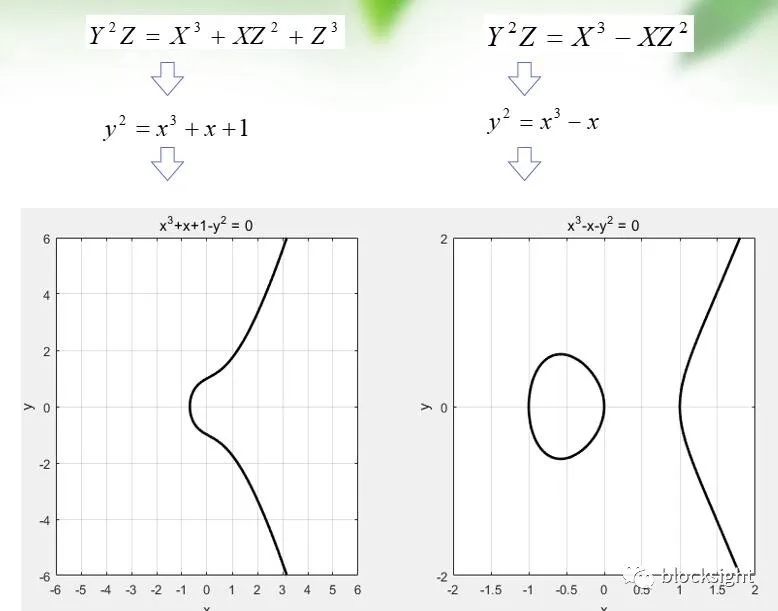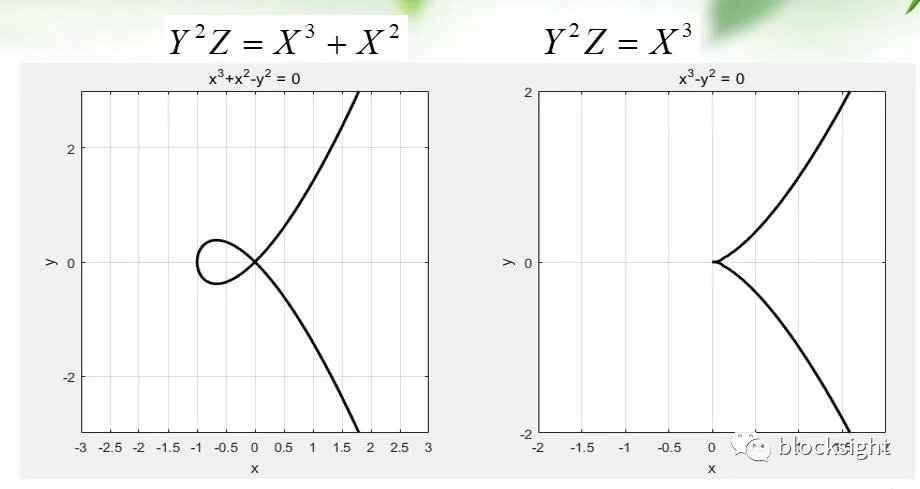# 区块链中的数学-椭圆曲线的背景及基本性质

https://www.interchains.cc/21445.html

## 射影平面区块链blockchain中的数学-椭圆曲线的背景及基本性质” />

## 射影平面坐标系

ax+by+c=0

aX+bY+cZ=0;

aX+bY+c1Z =0

（因为平行线斜率相同，故a,b 相同）。求两线交点，将两个方程联立，可得

c2Z= c1Z= -（aX+bY）

## 椭圆曲线方程

\$Y^2Z+a_1XYZ+a_3YZ^2=X^3+a_2x^2Z+a_4XZ^2+a_6Z^3\$

1.椭圆曲线方程是一个齐次方程

2.曲线上的每个点都必须是非奇异的（光滑的），偏导数FX(X,Y,Z)、FY(X,Y,Z)、FZ(X,Y,Z)不同为0

3.圆曲线的形状，并不是椭圆的。只是因为椭圆曲线的描述方程，类似于计算一个椭圆周长的方程故得名区块链blockchain中的数学-椭圆曲线的背景及基本性质” />区块链blockchain中的数学-椭圆曲线的背景及基本性质” />

\$y^2+a_1xy+a_3y=x^3+a_2x^2+a_4X+a_6\$